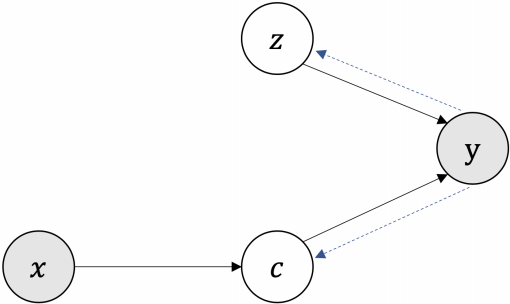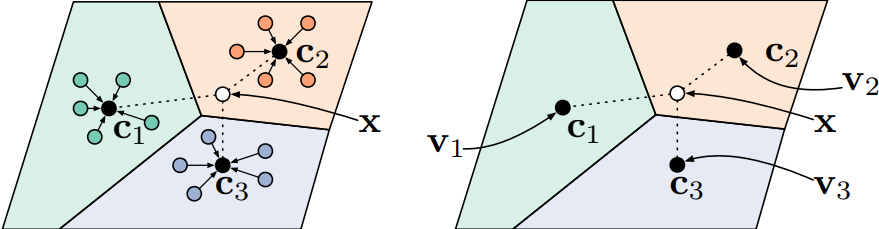ICLR 2020

Variational Template Machine for Data-to-Text Generation

• A graphical model for generating text $y$ from structured data $x$
• Similar to a variational autoencoder, but adds latent variables that represent a template $z$ and content $c$
• Continuous latent variables generate diverse output
• Reconstruction loss for output given data and template
• Template preserving loss: template variable can reconstruct the textThe graphical model of a Variational Template Machine. Ye et al.

Variational Inference

• Approximation for Bayesian inference with graphical models
• Computing posterior probabilities is hard because of the integration required to compute the evidence probability
• Approximates the posterior distribution using a variational posterior from a family of distributions
• Computing the KL divergence between the approximate and exact posterior would be equally hard, since it depends on the evidence probability
• Minimizing KL divergence is equal to minimizing the negative evidence lower bound (ELBO)
• ELBO is log evidence subtracted from the KL divergence
• The optimization problem is not as hard as integration

• Model consists of task layers and warp layers
• Task layers are updated using a normal loss
• Meta-learn warp-layers that modify the loss surface to be smoother
• Novelty: non-linear warp layers avoid dependence on the trajectory or the initialization, depending only on the task parameter updates at the current position of the search space

Meta-Learning

• Learning some parameters of the optimizer such as initialization
• Optimize for the final model accuracy as a function of the initialization
• When using gradient descent, the objective is differentiable
• Backpropagate through all training steps back into the initialization
• Vanishing / exploding gradients when the loss surface is very flat or steep
• Costly—usually scales to a handful of training steps only

Transformer-XH

• Adaptation of Transformer for structured text
• For example, multi-evidence reasoning: answer questions, following words that are linked to Wikipedia
• Extra hop attention attends to the first token of another sequence (representative of the entire sequence)

Deep Double Descent

• Bigger model means lower training loss
• At some point test error starts to increase, but with large enough models decreases again
• Occurs across various architectures (CNNs, ResNets, Transformers), data domains (NLP, vision), and optimizer (SGD, Adam)
• Also occurs when increasing training time
• In some cases increasing training data can hurt performance
• Effective model complexity takes training time into account

Towards Stabilizing Batch Statistics in Backward Propagation of Batch Normalization

• Unstable mean and variance estimation with too small batch sizes
• Batch renormalization (BRN): corrects batch mean and variance by moving average
• Moving average batch normalization: moving average of variance mean reduction + weight centralization

Compressive Transformer for Long-Range Sequence Modelling

• Train a language model on segments similar to Transformer-XL
• When moving to the next segment, the oldest N activations in the memory are compressed using a compressing function
• Lightweight and Dynamic Convolution: depth-wise separable convolution that runs in linear time
• Transformer-XL: train a language model on segments, but include activations from the previous segment in “extended context”
• Sparse Transformer: sparse attention masks
• Adaptive Attention Span: different attention heads can have longer or shorter spans of attention

A Closer Look at Deep Policy Gradients

• Policy gradient methods: optimize policy parameters to maximize the expected reward
• Variance reduction using baseline—separate the quality of the action from the quality of the state
• A canonical choice of baseline function is the value function
• Surrogate objective is a simplification of reward maximization used by modern policy gradient algorithms (policy is divided by the old policy, Schulman et al. 2015)
• Measure of gradient variance: mean pairwise correlation (similarity) between gradient samples
• Visualization of optimization landscapes with different number of samples per estimate

Laurent Dinh: Invertible Models and Normalizing Flows

• A model finds a representation $h = f(x)$ of a datapoint $x$ (in practice, an image)
• Generative models (VAE, GAN) can produce an image $x$ from its representation $h$
• GANs cannot encode images into the latent space
• VAEs support only approximate inference of latent variables from an image
• Normalizing flow is a sequence of invertible transformations
• Reversible generative models can encode an image into a latent space, making it possible to interpolate between two images

NICE

• Finds a representation such that $p(h)$ factorizes as $\prod p(h_d)$, where $h_d$ are independent latent variables (arguably a “good” representation)
• Prior distribution $p(h_d)$ is Gaussian or logistic
• Training: maximize the likelihood of the data using a “change of variables” formula
• $f(x)$ has to be invertible—achieved by splitting $x$ into $x_1$ and $x_2$ and using a transformation that transforms them into $x_1$ and $x_2 + m(x_1)$ respectively
• Sampling images: sample from $p(h)$ and use the inverse of $f(x)$

A Probabilistic Formulation of Unsupervised Text Style Transfer

• Change text style, keeping the semantic meaning unchanged
• Machine translation, sentiment transfer (positive ↔ negative)
• Unsupervised (only a nonparallel corpus)
• Previous work on text style transfer: autoencoding loss + adversarial loss using a language model as a discriminator
• Previous work on machine translation: cycle structures for unsupervised machine translation
• Novelty: probabilistic formulation of the above heuristic training strategies
• Translations of language A are “latent sequences” of language B and vice versa
• We want to utilize a language model prior within each language
• Train using variational inference

Estimating Gradients for Discrete Random Variables by Sampling without Replacement

• Strategies for obtaining gradients for discrete outputs: smoothening the outputs (relaxation), or sampling (REINFORCE)
• REINFORCE: move the gradient inside the expectation and estimate it using a sample
• With multiple samples one can use the average as a baseline
• Sampling without replacement can be done efficiently by taking the top $k$ of Gumbel variables
• Probability for sampling an unordered sample can be calculated as a sum over all possible permutations
• The estimator is changed to work with an unordered set

Reformer

• Memory efficiency: reversible residual layers, chunked FF and attention layers
• Time complexity: attention within buckets created using locality sensitive hashing

A Theoretical Analysis of the Number of Shots in Few-Shot Learning

• Prototypical networks cannot handle different numbers of shots between classes
• Performance drops when there’s a mismatch in the number of shots between meta-training and testing
• Trade-off between minimizing intra-class variance and maximizing inter-class variance is different when clustering a different number of embeddings
• Proposes an embedding space transformation

Prototypical Networks

• Aggregate experiences from learning other tasks to learn a few-shot task
• Form prototypes of each class as the average embedding of the labeled “support” examples
• Class likelihoods from the distances from the embedding of the current example to the prototypesPrototypical networks in the few-shot (left) and zero-shot (right) scenarios. Snell et al.

Mixed Precision DNNs

• Fixed-point representation for the weights and activations, with a different bitwidth for each layer
• A quantizer DNN is learned using gradient-based methods
• Which parameters (bitwidth, step size, minimum value, maximum value) to use for parameterization of uniform and power-of-two quantizations?
• The gradients with regard to the quantizer parameters are bounded and decoupled when choosing step size and maximum value, or minimum value and maximum value
• How to learn the parameters?
• A penalty term is added to the loss to enforce size constraints for the weights and activations

Training Binary Neural Networks with Real-to-Binary Convolutions

• Binary convolution can be implemented using fast xnor and pop-count operations
• Per-channel scaling is used to produce real-valued outputs
• Teacher-student with a real-valued teacher

On Mutual Information Maximization for Representation Learning

• Unsupervised learning based on information theoretic concepts
• InfoMax principle: a good representation should have high mutual information with the input
• MMI alone is not sufficient for representation learning, but modern methods work well in practice
• Multi-view approach: maximize mutual information between different views of the same input
• For example: split an image in half, encode both parts independently, and compare the mutual information between the parts
• If the representation encodes high-level features of the image, mutual information will be high; if it encodes noise, mutual information will be low

A Mutual Information Maximization Perspective of Language Representation Learning

• Many language tasks can be formulated as maximizing an objective function that is a lower bound on mutual information between different parts of the text sequence
• BERT (masked LM): word and corrupted word context, or sentence and following sentence
• Skip-gram (word2vec): word and word context
• InfoWorld (proposed by the authors): sentence and n-gram, both encoded using Transformer

Mutual Information Neural Estimation

• Mutual information: the amount the uncertainty about $X$ is reduced by knowing the value of $Z$
• Maximizing mutual information directly is infeasible
• The mutual information between $X$ and $Z$ can be expressed as the KL divergence between the joint probability distribution and the product of the marginal distributions: $I(X;Z) = D_{KL}(P_{XZ} \parallel P_X P_Z)$
• $E_P[f(x)] - \log E_Q[e^{f(x)}]$, where $f(x)$ is any real-valued function for which the expectations are finite, is always less than or equal to the KL divergence between $P$ and $Q$
• Donsker-Varadhan representation for KL divergence: supremum of this lower bound is equal to the KL divergence
• In theory, any function can be represented with a neural network ⇒ we can train a neural network $f(x)$ to maximize the lower bound

InfoNCE

• Maximize mutual information between target $x$ and context $c$
• One positive sample from $p(x \mid c)$ and $N-1$ negative samples from $p(x)$
• Loss based on noise contrastive estimation

ALBERT

• Small “sandwich” layer reduce the number of embedding parameters
• By default shares all parameters between layers
• Dropout removed
• More data

Incorporating BERT into Neural Machine Translation

• Initialize weights of NMT encoder from BERT (degradation)
• Initialize encoder and decoder weights with cross-lingual BERT trained on a multilingual corpus (small improvement)
• Create embeddings using BERT (significant improvement)
• BERT-fused NMT: additional attention to BERT (whose parameters are fixed) in each layer
• Drop-net trick: with certain probability perform a regularization step—use only BERT-encoder attention or self-attention
• SOTA results in semi-supervised NMT

Mixout

• Weight decay towards previous model parameters prevents catastrophic forgetting on the pretrained task
• Mixout sets parameters from a randomly selected neuron to those of the pretrained model during fine-tuning
• Corresponds to adaptive weight decay towards the pretrained model

Network Deconvolution

• There is a lot of correlation between nearby pixels, even when an image is not blurred
• Correlation in data causes gradient descent to take more steps
• Correlation between dimensions can be removed with a coordinate transform
• Calculate the correlation at every layer and apply inverse filtering
• Results in a sparse representation

A Signal Propagation Perspective for Pruning Neural Networks at Initialization

• By repeatedly training and pruning connections, model size can be reduced
• Even randomly initialized networks can be pruned prior to training, based on connection sensitivity
• It is unclear why pruning the initialization is effective
• Scaling of the initialization can have a critical impact

• Simultaneous translation: start translating before reading the full input
• Monotonic attention: stepwise probability for decision whether to read a source token or write a target token
• State of the art: Monotonic Infinite Loopback Attention (based on LSTM)
• Novelty: Transformer with multihead monotonic attention
• Independent stepwise probabilities for different heads